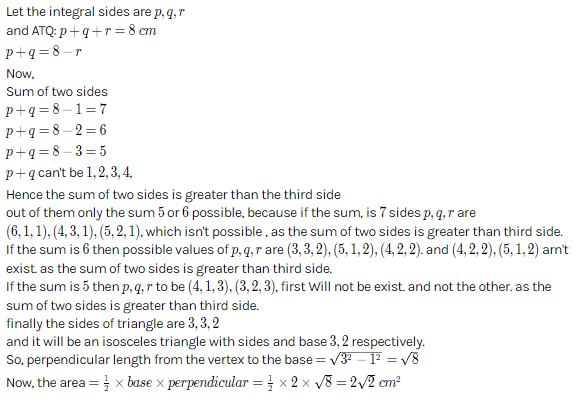×#### Thank you for registering.

One of our academic counsellors will contact you within 1 working day.

Click to Chat

1800-1023-196

+91-120-4616500

CART 0

• 0

MY CART (5)

Use Coupon: CART20 and get 20% off on all online Study Material

ITEM
DETAILS
MRP
DISCOUNT
FINAL PRICE
Total Price: Rs.

There are no items in this cart.
Continue Shopping
```
if T n =sin n theta+cos n theta ,prove that 6T 10 -15T 8 +10T 6 -1=0

```
11 years agoSaurabh Koranglekar
10233 Points
``````
8 months ago
```							Dear student T1=sinX+cosX; T2=1; T3=(sinX)^3+(cosX)^3, etc. Let s=sinX, c=cosX:T10=s^10+c^10; T8=s^8+c^8; T6=s^6+c^6, so:6s^10+6c^10-15s^8-15c^8+10s^6+10c^6-1=6s^10+6(1-s^2)^5-15s^8-15(1-s^2)^4+10s^6+10(1-s^2)^3-1=[6s^10+6(1-5s^2+10s^4-10s^6+5s^8-s^10)]+[-15s^8-15(1-4s^2+6s^4-4s^6+s^8)]+......[10s^6+10(1-3s^2+3s^4-s^6)]-1=-30s^8+6(1-5s^2+10s^4-10s^6+5s^8)-15(1-4s^2+6s^4-4s^6)+10(1-3s^2+3s^4)-1=-30s^8+30s^8+(6-15+10-1)+s^2(-30+60-30)+s^4(60-90+30)+s^6(-60+60)=0 QEDGood Luck
```
8 months ago
Think You Can Provide A Better Answer ?

## Other Related Questions on Trigonometry

View all Questions »### Course Features

• 731 Video Lectures
• Revision Notes
• Previous Year Papers
• Mind Map
• Study Planner
• NCERT Solutions
• Discussion Forum
• Test paper with Video Solution### Course Features

• 31 Video Lectures
• Revision Notes
• Test paper with Video Solution
• Mind Map
• Study Planner
• NCERT Solutions
• Discussion Forum
• Previous Year Exam Questions